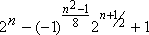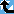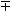# Gaussian Mersenne norm

The Prime Pages keeps a list of the 5000 largest known primes, plus a few each of certain selected archivable forms and classes. These forms are defined in this collection's home page.

Recall that the Mersenne primes are the primes of the form 2n-1. There are no primes of the form bn-1 for any other positive integer b because these numbers are all divisible by b-1. This is a problem because b-1 is not a unit (that is, it is not +1 or -1, the divisors of 1).

But what if we switch to the Gaussian integers, are there any Gaussian Mersenne primes? That is, are there any Gaussian primes of the form bn-1? If so, then b-1 would have to be a unit. The Gaussian integers have four units: 1, -1, i, and ±i. If b-1 is 1, then we get the usual Mersenne primes. If b-1 = -1, then bn-1 is -1, so there are no primes here! Finally if b-1 = ±i, then we get the conjugate pairs of numbers (1 ±i) n-1 with normsand these can be prime!

It is easy to show that a Gaussian integer a+bi is a Gaussian prime if and only if its norm

N(a + bi) = a2+b2

is prime or b=0 and a is a prime congruent to 3 (mod 4). For example, the prime factors of two are 1+i and 1-i, both of which have norm 2. So we have the following result:

Theorem. (1-i)n-1 is Gaussian Mersenne prime if and only if n is 2, or n is odd and the normis a rational prime.

These norms have been repeatedly studied as part of the effort to factor 2n-1 because they occur as factors in Aurifeuillian factorization

24m-2 + 1 = (22m-1 + 2m + 1) (22m-1 - 2m + 1).

So the first 23 examples of Gaussian Mersennes norms can be found in table 2LM of [BLSTW88], 21 of these were known by the early 1960's. These correspond to the Gaussian Mersenne primes (1 - i)n-1 for the following values of n:

2, 3, 5, 7, 11, 19, 29, 47, 73, 79, 113, 151, 157, 163, 167, 239, 241, 283, 353, 367, 379, 457, 997.

Much earlier, the mathematician Landry devoted a good part of his life to factoring 2n+1 and finally found the factorization of 258+1 in 1869 (so he was essentially the first to find the Gaussian Mersenne with n=29). Just ten years later, Aurifeuille found the above factorization, which would have made Landry's massive effort trivial [KR98, p. 37]! In all the Cunningham project's papers and books, beginning with [CW25], these Gaussian Mersenne norms have assumed a major role.

Mike Oakes, who apparantly originated the approach we used above in the early 1970's, has recently extended the list of known Gaussian Mersennes dramatically. We now know (1-i)n-1 is prime for the following values of n:

2, 3, 5, 7, 11, 19, 29, 47, 73, 79, 113, 151, 157, 163, 167, 239, 241, 283, 353, 367, 379, 457, 997, 1367, 3041, 10141, 14699, 27529, 49207, 77291, 85237, 106693, 160423 and 203789.
Gaussian Mersennes share many properties with the regular Mersennes and Oakes suggests they occur with the same density.

###Record Primes of this Type

rankprime digitswhowhencomment
1215317227 + 27658614 + 1 4610945 L5123 Jul 2020 Gaussian Mersenne norm 41?, generalized unique
224792057 - 22396029 + 1 1442553 L3839 Apr 2014 Gaussian Mersenne norm 40, generalized unique
323704053 + 21852027 + 1 1115032 L3839 Sep 2014 Gaussian Mersenne norm 39, generalized unique
421667321 - 2833661 + 1 501914 L137 Jan 2011 Gaussian Mersenne norm 38, generalized unique
521203793 - 2601897 + 1 362378 L192 Sep 2006 Gaussian Mersenne norm 37, generalized unique
62991961 - 2495981 + 1 298611 x28 Nov 2005 Gaussian Mersenne norm 36, generalized unique
72364289 - 2182145 + 1 109662 p58 Jun 2001 Gaussian Mersenne norm 35, generalized unique
82203789 + 2101895 + 1 61347 O Sep 2000 Gaussian Mersenne norm 34, generalized unique
92160423 - 280212 + 1 48293 O Sep 2000 Gaussian Mersenne norm 33, generalized unique
102106693 + 253347 + 1 32118 O Sep 2000 Gaussian Mersenne norm 32, generalized unique
11285237 + 242619 + 1 25659 x16 Aug 2000 Gaussian Mersenne norm 31, generalized unique
12277291 + 238646 + 1 23267 O Sep 2000 Gaussian Mersenne norm 30, generalized unique
13249207 - 224604 + 1 14813 x16 Jul 2000 Gaussian Mersenne norm 29, generalized unique
14227529 - 213765 + 1 8288 O Sep 2000 Gaussian Mersenne norm 28, generalized unique
15214699 + 27350 + 1 4425 O Sep 2000 Gaussian Mersenne norm 27, generalized unique
16210141 + 25071 + 1 3053 O Sep 2000 Gaussian Mersenne norm 26, generalized unique

###References

BLS75
J. Brillhart, D. H. Lehmer and J. L. Selfridge, "New primality criteria and factorizations of 2m ± 1," Math. Comp., 29 (1975) 620--647.  MR 52:5546 [The article for the classical (n2 -1) primality tests. Table errata in [Brillhart1982]]
BLSTW88
J. Brillhart, D. H. Lehmer, J. L. Selfridge, B. Tuckerman and S. S. Wagstaff, Jr., Factorizations of bn ± 1, b=2,3,5,6,7,10,12 up to high powers, Amer. Math. Soc., 1988.  Providence RI, pp. xcvi+236, ISBN 0-8218-5078-4. MR 90d:11009 (Annotation available)
Chamberland2003
Chamberland, Marc, "Binary BBP-formulae for logarithms and generalized Gaussian-Mersenne primes," Journal of Integer Sequences, 6:Article 03.3.7 (2003) 1--10.
CW25
A. J. C. Cunningham and H. J. Woodall, Factorizations of yn1, y = 2, 3, 5, 6, 7, 10, 11, 12 up to high powers (n), Hodgson, 1925.  London,
HS76
M. Hausmann and H. Shapiro, "Perfect ideals over the gaussian integers," Comm. Pure Appl. Math., 29:3 (1976) 323--341.  MR 54:12704
KR98a
R. Kumanduri and C. Romero, Number theory with computer applications, Prentice Hall, 1998.  Upper Saddle River, New Jersey,
McDaniel73
W. McDaniel, "Perfect Gaussian integers," Acta. Arith., 25 (1973/74) 137--144.  MR 48:11034
PH2002
Perschell, Karaloine and Huff, Loran, "Mersenne primes in imaginary quadratic number fields," (2002) avaliable from http://www.utm.edu/staff/caldwell/preprints/kpp/Paper2.pdf. (Abstract available)
Spira61
R. Spira, "The complex sum of divisors," Amer. Math. Monthly, 68 (1961) 120--124.  MR 26:6101